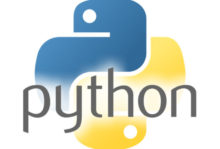# Python Lambda```Example of a definition to a lambda code :
def func1(x, y):
return x + y

##lambda would be :
result = lambda x, y: x + y
or
(lambda x, y: x + y)(value1,value2)```